# ML Aggarwal Class 10 Solutions for ICSE Maths Chapter 6 Factorization Chapter Test

## ML Aggarwal Class 10 Solutions for ICSE Maths Chapter 6 Factorization Chapter Test

ML Aggarwal Class 10 Solutions for ICSE Maths Chapter 6 Factorization Chapter Test

Question 1.
Find the remainder when 2x3 – 3x2 + 4x + 7 is divided by
(i) x – 2
(ii) x + 3
(iii) 2x + 1
Solution: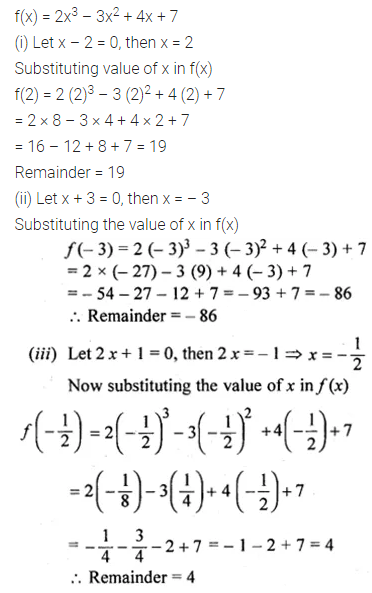Question 2.
When 2x3 – 9x2 + 10x – p is divided by (x + 1), the remainder is -24.Find the value of p.
Solution: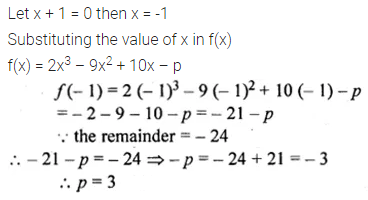Question 3.
If (2x – 3) is a factor of 6x2 + x + a, find the value of a. With this value of a, factorize the given expression.
Solution: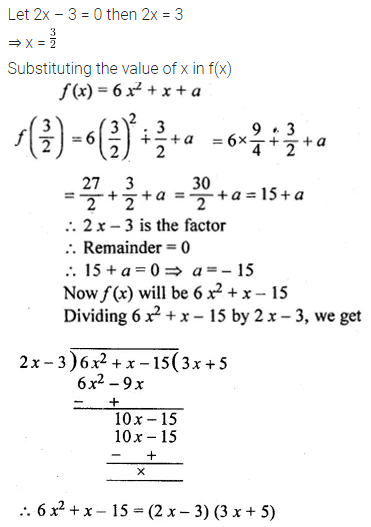Question 4.
When 3x2 – 5x + p is divided by (x – 2), the remainder is 3. Find the value of p. Also factorise the polynomial 3x2 – 5x + p – 3.
Solution: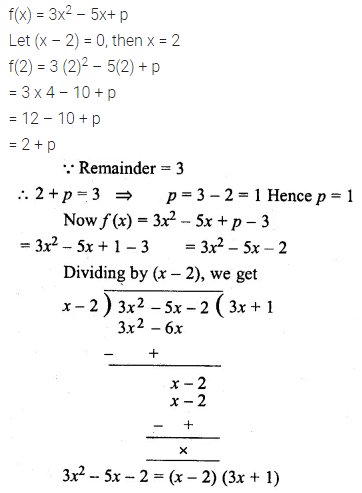Question 5.
Prove that (5x + 4) is a factor of 5x3 + 4x2 – 5x – 4. Hence factorize the given polynomial completely.
Solution: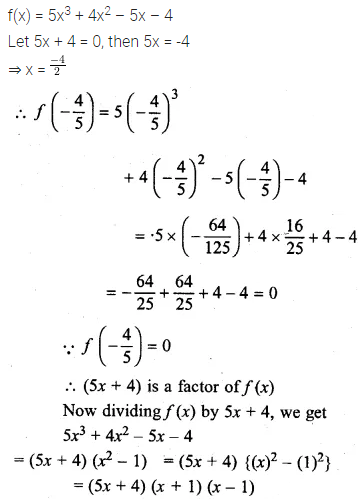Question 6.
Use factor theorem to factorise the following polynomials completely:
(i) 4x3 + 4x2 – 9x – 9
(ii) x3 – 19x – 30
Solution: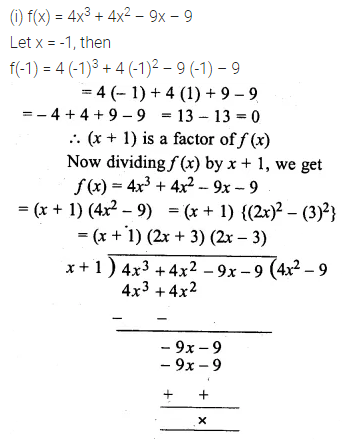Question 7.
If x3 – 2x2 + px + q has a factor (x + 2) and leaves a remainder 9, when divided by (x + 1), find the values of p and q. With these values of p and q, factorize the given polynomial completely.
Solution: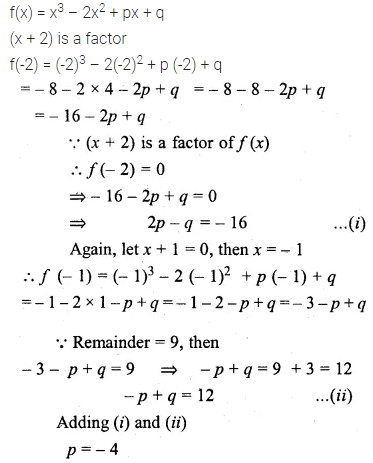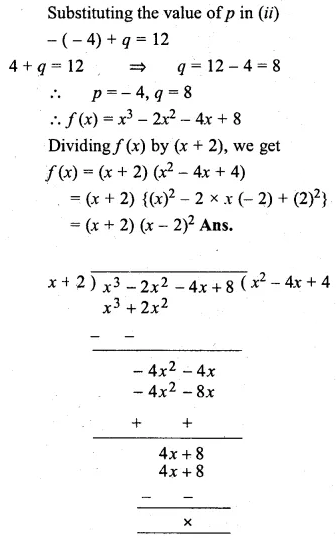Question 8.
If (x + 3) and (x – 4) are factors of x3 + ax2 – bx + 24, find the values of a and b: With these values of a and b, factorize the given expression.
Solution: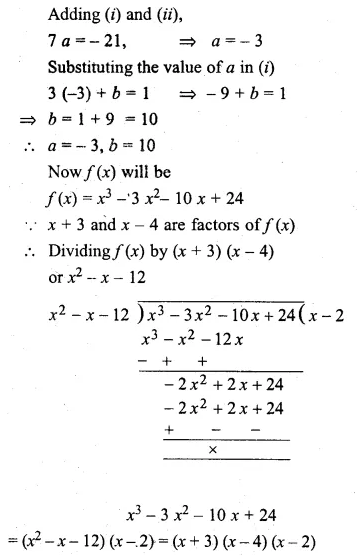Question 9.
If 2x3 + ax2 – 11x + b leaves remainder 0 and 42 when divided by (x – 2) and (x – 3) respectively, find the values of a and b. With these values of a and b, factorize the given expression.
Solution: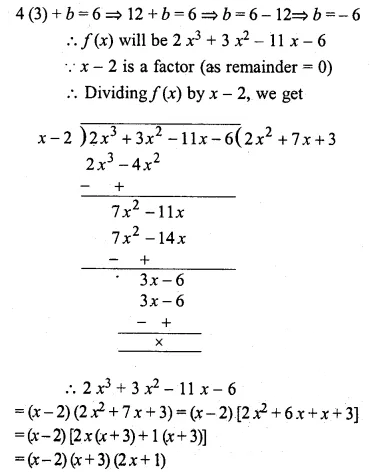Question 10.
If (2x + 1) is a factor of both the expressions 2x2 – 5x + p and 2x2 + 5x + q, find the value of p and q. Hence find the other factors of both the polynomials.
Solution: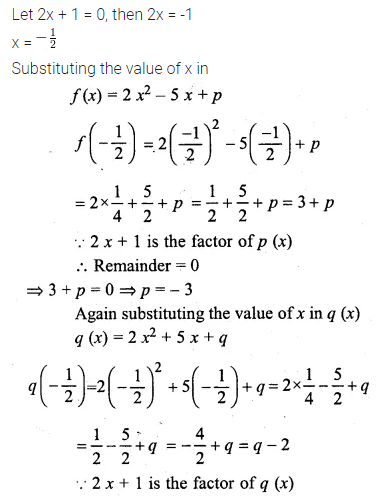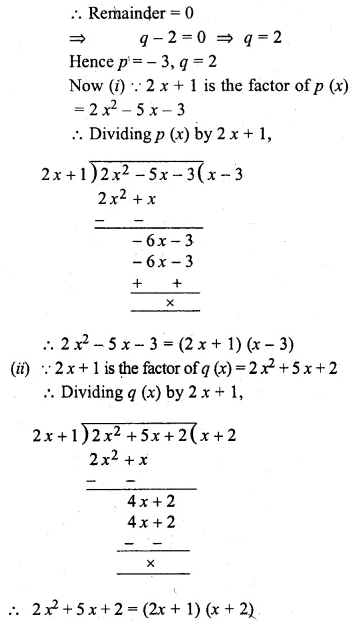Question 11.
When a polynomial f(x) is divided by (x – 1), the remainder is 5 and when it is,, divided by (x – 2), the remainder is 7. Find – the remainder when it is divided by (x – 1) (x – 2).
Solution:ML Aggarwal Class 10 Solutions for ICSE Maths